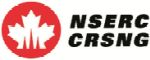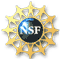# THEMATIC PROGRAMSJune 21, 2021January-June 2012 (Winter/Spring )
Thematic Program on Galois Representations

## April 23-27, 2012 Workshop on the p-adic Langlands program: recent developments and applications

Organizers: Christophe Breuil (Paris 11), Toby Gee (Imperial College, London),
Florian Herzig (U Toronto), Jan Nekovar (Paris 6)Supported by the National Science Foundation Award #1101503
 Abstracts
Abstracts

Noriyuki Abe, Hokkaido University
On a structure of modulo p compact induction of split p-adic groups

We consider a compact induction from a modulo p irreducible representation of a hyperspecial maximal compact subgroup of a split p- adic group. This representation is related to a parabolic induction. As an application, we give a classification of modulo p irreducible admissible representations in terms of supercuspidal representations.

Konstantin Ardakov, Queen Mary University of London
Localisation of affinoid enveloping algebras

I will explain how to relate representations of affinoid enveloping algebras to Berthelot's arithmetic D-modules on the flag variety, and sketch some applications to the p-adic representation theory of semisimple compact p-adic Lie groups.

Joël Bellaïche, Brandeis University
On Hecke algebras modulo $p$

We will present, motivate, and discuss special cases of a conjecture on the dimension and structure of the local components of the algebra of Hecke operators acting on the space of modular forms modulo a prime $p$ of a fixed level and all weights.

Francesc Castella, McGill University
On the p-adic Euler system of big Heegner points

Attached to a newform f of weight 2 and an imaginary quadratic field K, the Kummer images of Heegner points give rise to an anticyclotomic Euler System for the p-adic Galois representation associated with f. In this talk I will try to explain, assuming that the prime p splits in K, how the extension by Ben Howard of this construction to Hida families can be seen as producing a two-variable p-adic L-function in the spirit of Perrin-Riou. In the weight variable, we can thus show that Howard's construction interpolates the étale Abel-Jacobi images of Heegner cycles; in the anticyclotomic variable, this leads to new cases of the Bloch-Kato conjecture. The extension of some of these results to general CM fields in which p splits completely seems to be within reach.

Samit Dasgupta, University of California - Santa Cruz
$p$-arithmetic cohomology and completed cohomology of totally indefinite Shimura varieties

This is a report on work in progress with Matthew Greenberg. Let $F$ be a totally real field and let $p$ be a rational prime that for simplicity we assume is inert in $F$. Let $f$ be a modular form for a totally indefinite quaternion algebra $A$ over $F$ whose local representation at $p$ is Steinberg. We define a Darmon $\mathcal{L}$-invariant attached to $f$, which is a vector of $p$-adic numbers indexed by the embeddings of $F$ into $\mathbf{C}_p$. This $\mathcal{L}$-invariant is defined using the cohomology of a $p$-arithmetic subgroup of $A^*$ and is modeled after Darmon's definition of the $L$-invariant in the case
$F=\mathbf{Q}, A=\mathrm{M}_2(\mathbf{Q})$.

Next we consider certain $p$-adic Banach space representations of $\mathrm{GL}_2(F_p)$ denoted $B(k,\mathcal{L})$, where $k$ is the vector of weights of $f$ and $\mathcal{L}$ is a vector as before. These Banach space representations generalize the construction of Breuil in the case $F=\mathbf{Q}$, and build on work of Schraen. Our main result is that there exist $\mathrm{GL}_2(F_p)$-interwining operators from $B(k,\mathcal{L})$ to the $f$-isotypic component of the completed cohomology of $A$ if and only if the vector $\mathcal{L}$ is the negative of the Darmon $\mathcal{L}$-invariant. This generalizes a result of Breuil in the case $F=\mathbf{Q}, A=\mathrm{M}_2(\mathbf{Q})$.

Gabriel Dospinescu, École Polytechnique
Extensions of de Rham representations and locally algebraic vectors

In this joint work with Vytautas Paskunas, we extend Colmez' results on locally algebraic vectors in the p-adic Langlands correspondence. The main result is the following: if p>3 and if Pi is a finite length admissible unitary Banach space representation of GL_2(Q_p), for which the locally algebraic vectors are dense in Pi, then the image of Pi by the Montreal functor is a potentially semi-stable p-adic Galois representation. We will explain how the theory of phi-gamma modules can be applied to prove this theorem.

Matthew Emerton, University of Chicago
Density of Galois representations having prescribed types

If X is a deformation space of p-adic Galois representations (either local or global), and S is a set of types, then one can consider the subset X(S) of points in the rigid analytic generic fibre of X whose associated Galois representations are potentially Barsotti--Tate at p of type lying in the set S. We give conditions on the set S which imply that the set X(S) is Zariski dense in X. As one application we conclude that the set of representations which are both potentially Barsotti--Tate and crystabelline at p is Zariski dense in X. This is joint work with Vytautus Paskunas.

Laurent Fargues, Université de Strasbourg
Beyond the curve

I will give results and conjectures I never had time to speak about concerning the curve I defined with J.M.-Fontaine.

Toby Gee, Imperial College
The Breuil-Mézard Conjecture for potentially Barsotti-Tate representations

I will discuss the proof of many cases of the Breuil-Mézard conjecture for two-dimensional potentially Barsotti-Tate representations (joint with Mark Kisin).

David Geraghty, Princeton University
Modularity lifting in non-regular weight

Modularity lifting theorems were introduced by Taylor and Wiles and formed a key part of the proof of Fermat's Last Theorem. Their method has been generalized successfully by a number authors but always with the restriction that the Galois representations and automorphic representations in questions have regular weight. I will describe a method to overcome this restriction in certain cases. I will focus mainly on the case of weight 1 elliptic modular forms. This is joint work with Frank Calegari.

Florian Herzig, University of Toronto
Ordinary representations of GLn(Qp) and fundamental algebraic representations

Motivated by a hypothetical p-adic Langlands correspondence for GLn(Qp) we associate to an n-dimensional ordinary (i.e. upper-triangular) representation rho of Gal(Qp-bar/Qp) over E a unitary Banach space representation Pi(rho)^ord of GLn(Qp) over E that is built out of principal series representations. (Here, E is a finite extension of Qp.) There is an analogous construction over Fp-bar. In the latter case we show under suitable hypotheses that Pi(rho)^ord occurs in the rho-part of the cohomology of a compact unitary group. This is joint work with Christophe Breuil.

Payman Kassaei, King's College London
Modularity lifting in weight (1,1,...,1)

We show how p-adic analytic continuation of overconvergent Hilbert modular forms can be used to prove modularity lifting results in parallel weigh one. Combined with mod-p modularity results, these results can be used to prove certain cases of the strong Artin conjecture over totally real fields.

Ruochuan Liu, University of Michigan
Crystalline periods of eigenfamilies of p-adic representations

We will explain a (phi,Gamma)-module variant of Kisin's finite slope subspace technique and its applications to eigenfamilies of p-adic representations

Jonathan Pottharst, Boston University
Triangulation of eigenvarieties

In the 1980s, Wiles showed the Galois representation over a Hida family to be reducible when restricted to a decomposition group at p. This result is the basis for the study of variation of Selmer groups of modular forms in the family. In joint work with K. S. Kedlaya and L. Xiao, we prove the analogous result over the eigencurve, using a strong finiteness result for Galois cohomology of rigid analytic families of (phi,Gamma)-modules over the Robba ring. Applications to Iwasawa theory are then possible by our previous work. (A similar result has been found recently by R. Liu, using a significant strengthening of Kisin's method of interpolation of crystalline periods.)

David Savitt, University of Arizona
The Buzzard-Diamond-Jarvis conjecture for unitary groups

We will discuss the proof of the weight part of Serre's conjecture for rank two unitary groups in the unramified case (that is, the Buzzard-Diamond-Jarvis conjecture for unitary groups).  This is joint work with Toby Gee and Tong Liu.  More precisely, we prove that any Serre weight which occurs is a predicted weight; this completes the analysis begun by Barnet-Lamb, Gee, and Geraghty, who proved that all predicted weights occur.  Our methods are purely local, using Liu's theory of (phi,G-hat) modules to determine the possible reductions mod p of certain two-dimensional crystalline Galois representations.

Benjamin Schraen, Centre national de la recherche scientifique
On the presentation of supersingular representations

Let F be a quadratic extension of the field of p-adic numbers and k an algebraic closed field of characteristic p. We say that a smooth representation of GL2(F) on a k-vector space is of finite presentation if it is a of finite presentation in the category of smooth representations of GL2(F). In this talk we prove that if F is different of Qp, irreducible supersingular representations of GL2(F) on k-vector spaces are not of finite presentation.

Claus Sorensen, Princeton University
Eigenvarieties and invariant norms

By a slight modification of the classical local Langlands correspondence, one can attach a locally algebraic representation of GL(n) to any n-dimensional potentially semistable Galois representation (with distinct Hodge-Tate weights). A conjecture of Breuil and Schneider asserts that the former admits an invariant norm. We will prove this when the latter comes from a classical point on an eigenvariety. More generally, for any definite unitary group G, we will explain how its eigenvariety (of some fixed tame level) mediates part of a global correspondence between Galois representations of the CM field, and Banach-Hecke modules B with a unitary G-action. For any regular weight W, we express the locally W-algebraic vectors of B in terms of the Breuil-Schneider representation on the Galois side.

Yichao Tian, Morningside Center of Mathematics
Analytic continuation of weight 1 overconvergent Hilbert modular forms in the tamely ramified case

Abstract: The method of analytic continuation was initiated by Buzzard-Taylor to treat the icosahedral case of the Artin conjecture over Q. In this talk, I will explain how to extend this approach to the Hilbert case. Let p be an odd prime number, and F be a totally real field in which p is unramified. We prove that a p-adic Galois representation over F, which is residually ordinarily modular and saitsifies certain local conditions at p, comes from actually a Hilbert modular form of weight 1. For the moment, we only know how to treat the case where the Galois representation is tamely ramified at p. This is a joint work with Payman Kassaei and Shu Sasaki. I hope Payman will have explained the general principle, then I will focus on the details of the analytic continuation process.

Gergely Zábrádi, Eötvös Loránd
From (phi,Gamma)-modules to G-equivariant sheaves on G/P

Let G be the Q_p-points of a Q_p-split connected reductive group with Borel subgroup P=TN. For any simple root alpha of T in N, we associate functorially to a finitely generated etale (phi,Gamma)-module D over Fontaine's ring (equipped with an additional linear action of the group Ker(alpha)) a G-equivariant sheaf on the flag variety G/P. This functor is faithful. In case of G=GL_2(Q_p) the global sections of this sheaf coincide with the representation D\boxtimes P^1 constructed by Colmez. This is joint work with Peter Schneider and Marie-France Vigneras.

Back to Top